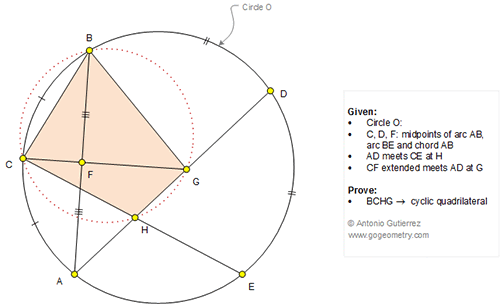## Wednesday, August 31, 2016

### Geometry Problem 1254: Circle, Arc, Chord, Midpoint, Cyclic Quadrilateral, Concyclic Point

Geometry Problem. Post your solution in the comment box below.
Level: Mathematics Education, High School, Honors Geometry, College.

This entry contributed by Sumith Peiris, Moratuwa, Sri Lanka.

Click the figure below to view more details of problem 1254.1.Join AE and form the triangle AHE.
Let Angle BAD = A => Angle DAE = Angle HAE = A (since D is midpoint of B & E)
Let Angle ABC = B => Angle CEA = Angle HEA = B => Angle AHE = Angle CHG = 180-(A+B)
and since C is midpoint of AB => Angle CAB = B and triangle ABC is isosceles
=> CF perpendicular to AB and ABG is isosceles => Angle ABG = A
Therefore Angle CBG = A+B & Angle CHG = 180-(A+B) => BCGH are concyclic

2.Problem 1254
Is <GBA=<GAB=<DAB (AF=FB, FG=perpendicular bisector of AB),<BAD=arcBD=arcDE.